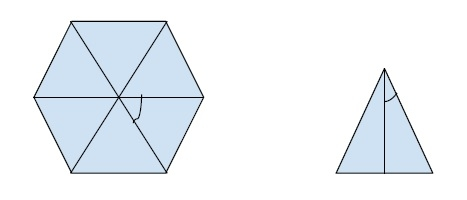# Area of a n-sided regular polygon with given side length in C++

In this problem for finding the area of an n-sided regular polygon with a given side, we will derive the formula for the area of the figure and create a program based on it. But before that let's revise the basics to understand the topic easily.

An N-sided regular polygon is a polygon of n side in which all sides are equal. For example regular pentagon, regular hexagon, etc.

The area is the quantitative representation of the extent of any two-dimensional figure.

To find the area of this figure we need to find the area of individual triangles in the figure and multiply it by the number of sides it has. Since we are given n sided.Now, from the above figure, we can create a formula for the area.

Each side of the regular polygon can create one triangle of side a (side of a polygon) and angle 180 / n (n is a number of sides of a polygon). So, the area can be found using the formula,

Area of triangle = ½ * b * h

Now, h = a * tan(180/n)

So , area = ½ * a * a / 2 * tan(180/n)
= a * a / (4 * tan(180/n))

Using this formula for an individual triangle of the polygon, we can create the area of the whole polygon,

Area of n-sided regular polygon = n * (a * a / (4 * tan(180 /n)))

## Algorithm

Step 1 : calculate the value of angle using (180 / n)
Step 2 : Calculate the area of regular polygon using n * (a * a / (4 * tan(180 /n))) .
Step 3 : Print the area of polygon.

## Example

Live Demo

#include<iostream>
#include<math.h>
using namespace std;
int main(){
float a = 12, n = 9;
float area=(a * a * n) / (4 * tan((180 / n) * 3.14159 / 180));
cout<<"The area of "<<n<<" sided regular polygon of side "<<a<<" is "<<area;
return 0;
}

## Output

The area of 9 sided regular polygon of side 12 is 890.183